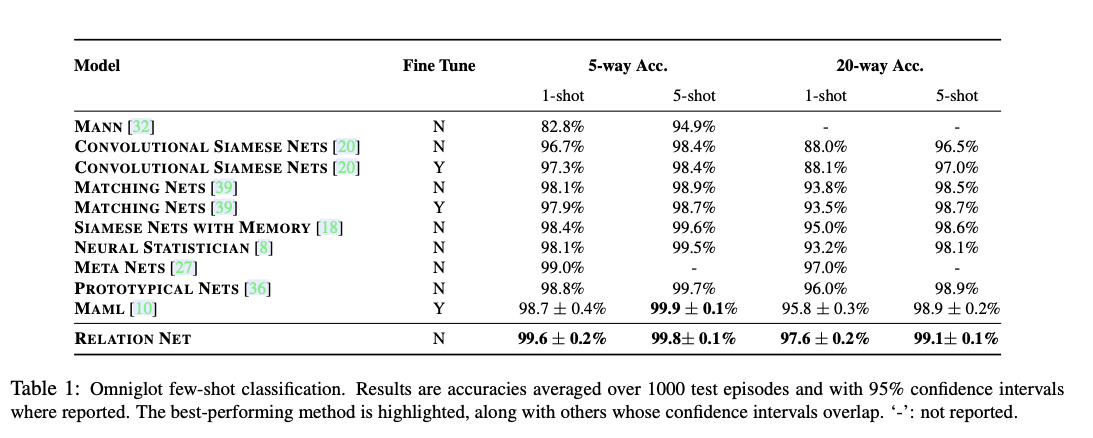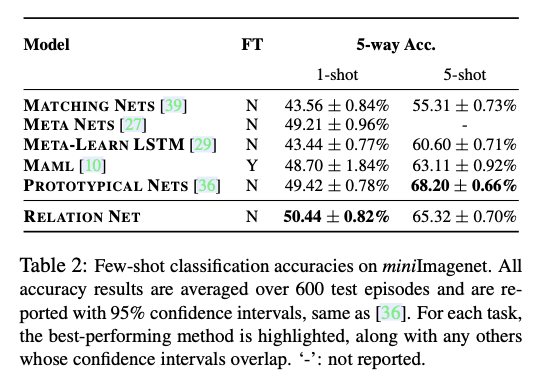FSL入门

0x01 什么是小样本学习

简单介绍

Few-Shot Learning 泛指从少量标注数据中学习的方法和场景，理想情况下，一个能进行 Few-Shot Learning 的模型，也能快速地应用到新领域上。Few-Shot Learning 是一种思想，并不指代某个具体的算法、模型，所以也并没有一个通用的、万能的模型，能仅仅使用少量的数据，就把一切的机器学习问题都解决掉，讨论 Few-Shot Learning 时，一般会聚焦到某些特定的问题上，比如 Few-Shot Learning 思想应用到分类问题上时就称之为 Few-Shot Classification。

(PS:以下提及的Few-shot learning主要针对分类任务，相关论文也是分类任务)

研究意义

（主动学习的初衷也是降低人工标记成本。但是与小样本学习的不同在于主动学习会有大量的原始未标记的样本，在学习的过程中选择信息量比较多的样本交予专家去标记。但是小样本学习可能因为种种原因无法获取足够的原始未标记数据，这对学习难度会更大。）

主要困难

• 现在已有一定量标注的数据集，数据集有很多种类，但是每一类的样本数量不多！现在可以将这数据集作为训练集进行训练，获得模型$M$.
• 给定一批新标注的数据集(与之前的训练集毫无交叉，换句话说，模型从未见过新数据)，其中共有$N$类，每一类有$k$个样本。（即$N$-way $k$-shot 问题）
• $k=1$时就是 one-shot
• $k=0$时就是 zero-shot
• 任务就是利用模型$M$实现对新数据集的分类。

（举个例子就是，你见过大量的鸡、大量的鸭，突然某天见到一只鹅，你不认识，别人告诉你这是鹅，你就会在心里记下 身体呈白色，体态像鸭的两只脚禽兽就是鹅。但是某天一只黑天鹅出现了，这是啥？！ ）

$R(h)=\int l(h(x),y)dp(x,y)=\mathbb{E}[l(h(x),y)] \tag{1}$

$R(h_{Train})=\frac{1}{n}\sum_{i=1}^{|Train|}l(h(x^{i}),y^{i}) \tag{2}$

(训练集的风险函数$R(h_{Train})$,可获得的最优值情况下的风险函数$R(\hat{h})$)

$\mathbb{E}[R(h_{Train})-R(h^*)]=\mathbb{E}[R(\hat{h})-R(h^*)]+\mathbb{E}[R(h_{Train})-R(\hat{h})] \tag{3}$

approximation error：只与算法L的假设空间$\mathcal{H}$相关（假设空间采样）。

estimation error：不但与假设空间$\mathcal{H}$相关，还与训练集的采样相关（训练样本采样）。

$\varepsilon(\mathcal{H},Train)\rightarrow\varepsilon(\mathcal{H},\infin)=\lim_{Train\rightarrow \infin}\mathbb{E}[R(h_{Train})-R(\hat{h})]=0$

0x02 解决小样本学习的主要方法

基本过程：

• 挖掘事物高层的语义表示，
• 得到先验知识(从任务集合中提取和传播可迁移的知识)，
• 使训练出的模型具有可迁移性(能迁移到小样本)。

主要方法：

• 数据增强：通过何种方式扩充数据样本的大小。
• 预估模型：挖掘高层次的语义特征，降低模型参数空间和优化难度，约束模型空间。
• 改进算法：传统的SGD算法不适于FSL，只能通过其他优化方式进行训练

Paper

0x00 Abstract

During meta-learning, it learns to learn a deep distance metric to compare a small number of images within episodes, each of which is designed to simulate the few-shot setting. Once trained, a RN is able to classify images of new classes by computing relation scores between query images and the few examples of each new class without further updating the network.

0x01 Introduction

Few-shot learning aims to recognise novel visual categories from very few labelled examples.

Data augmentation and regularisation techniques can alleviate overfitting in such a limited-data regime, but they do not solve it.

While promising, most existing few-shot learning approaches either require complex inference mechanisms, complex recurrent neural network (RNN) architectures, or fine-tuning the target problem.

Our approach is most related to others that aim to train an effective metric for one-shot learning.

By expressing the inductive bias of a deeper solution (multiple non-linear learned stages at both embedding and relation modules), we make it easier to learn a generalisable solution to the problem.

Specifically, we propose a two-branch Relation Network (RN) that performs few-shot recognition by learning to compare query images against few-shot labeled sample images.

• First an embedding module generates representations of the query and training images.
• Then these embeddings are compared by a relation module that determines if they are from matching categories or not.

Our proposed strategy also directly generalises to zero-shot learning. In this case the sample branch embeds a single-shot category description rather than a single exemplar training image, and the relation module learns to compare query image and category description embeddings.

Learning to Fine-Tune

• The successful MAML approach aimed to meta-learn an initial condition (set of neural network weights) that is good for fine-tuning on few-shot problems.
• However both of these approaches suffer from the need to fine-tune on the target problem.
• In contrast, our approach solves target problems in an entirely feed-forward manner with no model updates required, making it more convenient for low-latency or low-power applications.

RNN Memory Based

• Here the idea is typically that an RNN iterates over an examples of given problem and accumulates the knowledge required to solve that problem in its hidden activations, or external memory.
• These architectures face issues in ensuring that they reliably store all the, potentially long term, historical information of relevance without forgetting.
• In our approach we avoid the complexity of recurrent networks, and the issues involved in ensuring the adequacy of their memory. Instead our learning-to-learn approach is defined entirely with simple and fast feed forward CNNs.

Embedding and Metric Learning Approaches

• Another category of approach aims to learn a set of projection functions that take query and sample images from the target problem and classify them in a feed forward manner.
• Metric-learning based approaches aim to learn a set of projection functions such that when represented in this embedding, images are easy to recognise using simple nearest neighbour or linear classifiers.
• The most related methodologies to ours are the prototypical networks and the siamese networks.
• learning embeddings that transform the data such that it can be recognised with a fixed nearest-neighbour or linear classifier.
• our framework further defines a relation classifier CNN,can be seen as providing a learnable rather than fixed metric, or non-linear rather than linear classifier.

Zero-Shot Learning

Our approach is designed for few-shot learning, but elegantly spans the space into zero-shot learning (ZSL) by modifying the sample branch to input a single category description rather than single training image.

0x03 Methodology

1. Problem Definition

• 数据分为 训练集支持集测试集

The support set and testing set share the same label space, but the training set has its own label space that is disjoint with support/testing set.

If the support set contains K​ labelled examples for each of C unique classes, the target few-shot problem is called C-way K-shot.

Sample Set $\mathcal{S}$: 来自训练集

Query Set $\mathcal{Q}$:来自$\mathcal{S}$

• This sample/query set split is designed to simulate the support/test set that will be encountered at test time.
• A model trained from sample/query set can be further fine-tuned using the support set, if desired.
• In this work we adopt such an episode-based training strategy.

We aim to perform meta-learning on the training set, in order to extract transferrable knowledge that will allow us to perform better few-shot learning on the support set and thus classify the test set more successfully.

• 其整体框架如下图所示：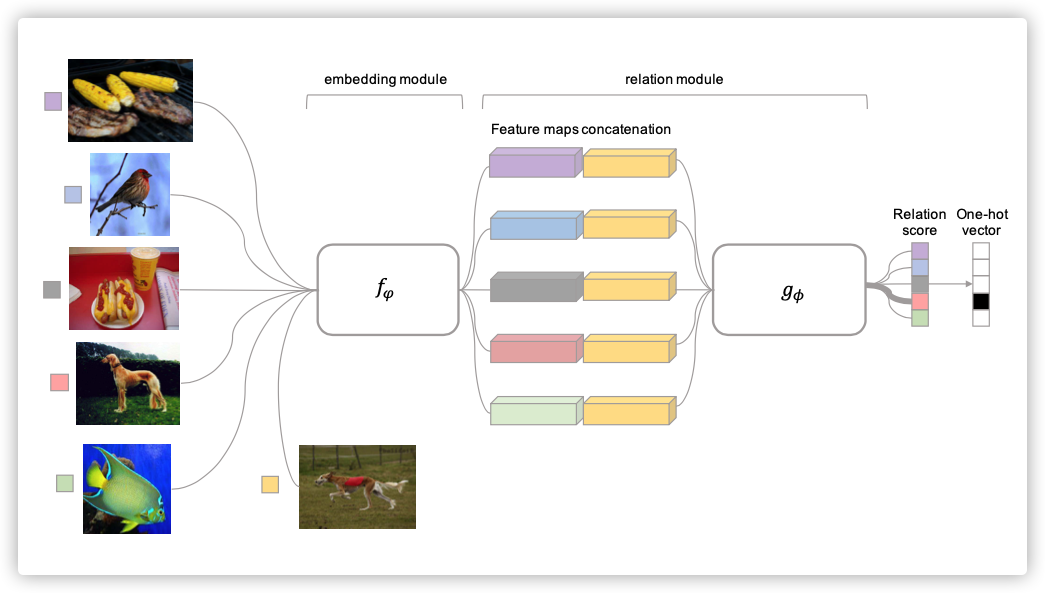2. Model

• embedding module$f_{\varphi}$

• combined operator: $\mathcal{C}(f_{\varphi}(x_i),f_{\varphi}(x_j))$

• relation module: $g_{\phi}$,输出为 relation score表示相似得分为0-1之间标量

• relation score$r_{i,j}=g_{\phi}(\mathcal{C}(f_{\varphi}(x_i),f_{\varphi}(x_j)))$

$One-shot$:最终输出就是单个 $f_{\varphi}$输出

$K-shot$:最终输出就是$K$$f_{\varphi}$输出和

Objective function

$\varphi,\phi \leftarrow \mathop{argmin}\limits_{\varphi,\phi}\sum_{i=1}^m\sum_{j=1}^n(r_{i,j}-\mathbf{1}(y_i==y_j))^2$

3. Zero-shot Learning

$r_{i,j}=g_{\phi}(\mathcal{C}(f_{\varphi_1}(v_c),f_{\varphi_2}(x_j)))$

4. Network Architecture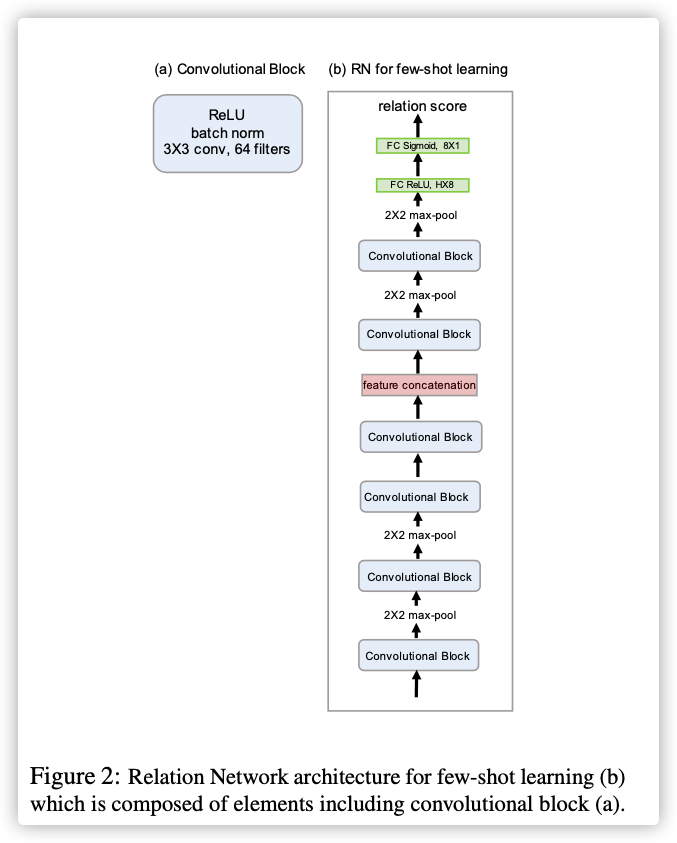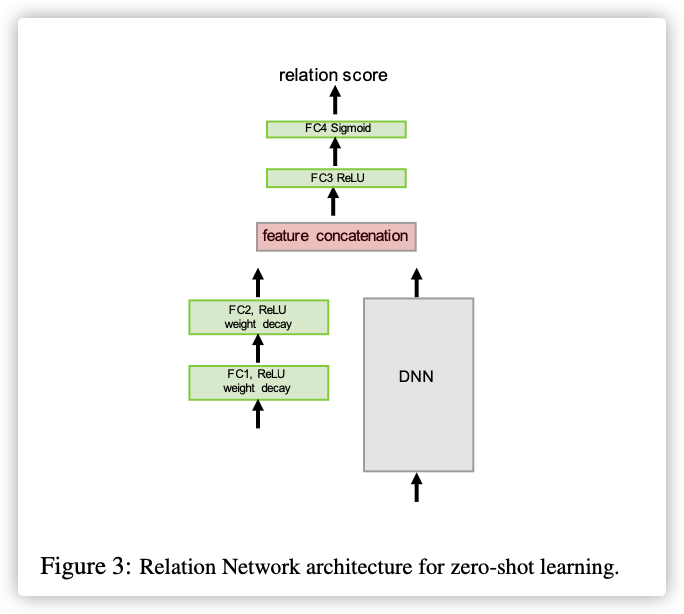0x04 Experiments